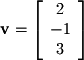The magnitude of an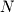-dimensional vector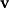with entriesis defined as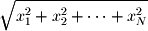The notation for this operation is either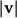or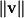. You might note that theis the identical symbol for absolute value of scalars. Indeed, a vector's magnitude is its absolute value away from the origin. One can think of vector magnitude as a generalization of absolute value to higher dimensions.
Excercise 2-3.
Find the magnitude of the vector